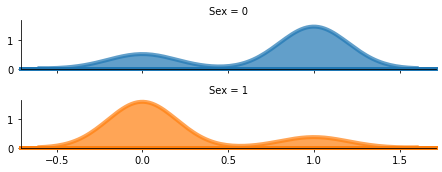# How To Make Ridgeline plot in Python with Seaborn?

Prerequisite: Seaborn

Ridgeline plot is a set of overlapped density plots that help in comparing multiple distributions among datasets. The Ridgeline plots look like a mountain range, they can be quite useful for visualizing changes in distributions over time or space. Sometimes it is also known as “joyplot”, in reference to the iconic cover art for Joy Division’s album Unknown Pleasures. In this article, We will see how to generate Ridgeline plots for the dataset.

### Installation

Like any another python library, seaborn can be easily installed using pip:

```pip install seaborn
```

This library is a part of Anaconda distribution and usually works just by import if your IDE is supported by Anaconda, but it can be installed too by the following command:

```conda install seaborn
```

#### Procedure

• Load the packages required to generate the Ridgeline plot with Python.
• Read the Dataset. In this example, we use the read_csv() method to load the dataset. In the given example we will only display the top 5 entries using the head() method.
• Generate RidgePlot. The Ridgeline Plot uses faceting meaning it creates small multiples, in a single column. To generate Ridgeline Plot Seaborn uses FacetGrid() method and all required information should be passed to it

Syntax: seaborn.FacetGrid(data, row, col, hue, palette, aspect, height)

Parameters:

1. data: Tidy (“long-form”) dataframe where each column is a variable and each row is an observation.
2. row, col, hue: Variables that define subsets of the data, which will be drawn on separate facets in the grid.
3. height: Height (in inches) of each facet.
4. aspect: Aspect ratio of each facet, so that aspect * height gives the width of each facet in inches.
5. palette: Colors to use for the different levels of the hue variable.
• Use the map() method to creates a density plot in each element of the grid. In this example, we need a density plot so use kdeplot() method which available in Seaborn.

Sample Database: Dataset used in the following example is downloaded from kaggle.com. The following link can be used for the same.

Database: titanic_train.csv

Example:

## Python3

 `import` `seaborn as sns ` `import` `matplotlib.pyplot as plt ` `import` `pandas as pd ` `from` `sklearn ``import` `preprocessing ` ` `  `df ``=` `pd.read_csv(``"titanic_train.csv"``) ` `df.dropna() ` ` `  `le ``=` `preprocessing.LabelEncoder() ` `df[``"Sex"``] ``=` `le.fit_transform(df[``"Sex"``]) ` ` `  ` `  `rp ``=` `sns.FacetGrid(df, row``=``"Sex"``, hue``=``"Sex"``, aspect``=``5``, height``=``1.25``) ` ` `  `rp.``map``(sns.kdeplot, ``'Survived'``, clip_on``=``False``, ` `       ``shade``=``True``, alpha``=``0.7``, lw``=``4``, bw``=``.``2``) ` ` `  `rp.``map``(plt.axhline, y``=``0``, lw``=``4``, clip_on``=``False``) `

Output :